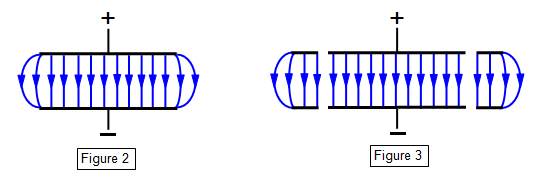# The parallel-plate capacitorIn its most basic form a capacitor is simply two metal plates with a material of permittivity e filling the space between them shown in Figure 1. Such an arrangement is called a parallel plate capacitor. The plates of a charged parallel capacitor each carry charges of the same size but of opposite sign.

These charges generally spread evenly over the surfaces of the plates but there is a build up of charge at the sharp edges of each plate. The effect of this on the field between the plates is shown on Figure 2. This effect can be reduced by using a 'guard ring'. (See Figure 3.) Even without a guard ring the field within a parallel plate capacitor is uniform except very close to the edges.Let the area of the plates be A and their separation d; let one plate have a charge +Q and the other -Q, and let the capacitance be C. Assume the field between the plates to be uniform and that the charge density is also uniform.

The charge density on the plates (sA) is Q/A and therefore the electric field intensity E between the plates is given by:

Electric field intensity (E) = s/e = QeA

But if V is the potential difference between the plates we have: E = V/d = Q/eA and therefore, since C = Q/V

Capacitance (C) = eA/d

Therefore the capacitance increases if the area of the plates is increased or their separation decreased. (See Experiments/Capacitor parallel plate)

You can also see that the insertion of a material with a high permittivity will increase the capacitance of a capacitor. An explanation of this is given in the file on dielectrics.
(Please refer to the section on Electric fields for an explanation of charge density and permittivity).

Example problems
1. Calculate the separation of the plates of an air filled capacitor of capacitance 25 nF if the plates have an area of 40 cm2. (Permittivity of free space (eo) = 8.85x10-12 Fm-1
Capacitance (C) = eoA/d = 25x10-9 = [8.85x10-12x40x10-4]/d
Therefore: d = 0.0014 mm

2. Calculate the capacitance of a pair of parallel plates of area 25 cm2 if they are separated by a piece of Perspex 0.1 mm thick. Take the relative permittivity of Perspex to be 3.5.

(Permittivity of free space (eo) = 8.85x10-12 Fm-1)
Capacitance = [8.85 x 10-12 x 3.5 x 25 x 10-4]/10-4 = 7.43 x 10-10 = 0.74 nF.

As explained in the file on capacitors an air filled parallel plate capacitor with plates 1 mm apart and with a capacitance of 1 F would have plates each of a tenth of a square kilometre!

## Changing the plate separation of a parallel plate capacitor

If we consider the formula for the parallel-plate capacitor we can see what happens as we change the plate separation. There are two different cases to consider:
(a) where the capacitor remains connected to the source of electrical potential, and
(b) where the capacitor is disconnected after the initial charge Q has been placed on the plates.

(a) The potential V remains constant and therefore the charge on the plates varies. This is because V = Q/C and C = eA/d. As the separation of the plates is increased the charge on them decreases to maintain a constant value of V. The electric field intensity between the plates therefore falls. The change in energy of the capacitor is explained by the movement of charges in the wires as the capacitor partly discharges to maintain the potential.

(b) If the supply is disconnected, however, then it is the charge on the plates that remains constant and the potential difference between them increases as the plates are separated. The electric field intensity remains constant in this case. This can be explained by the fact that work has to be done on the plates to separate them. There is an increase in the energy of the capacitor which can explained by the addition of energy to moving the plates apart against their mutual attraction.